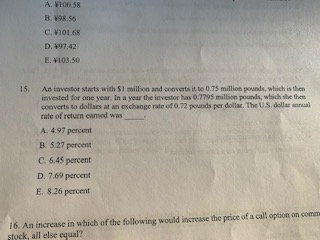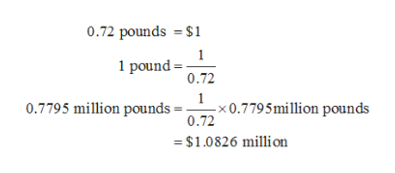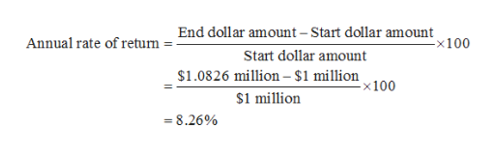# A. VI00.58B. V98.55C. VI01.68D. 497.42E. V103.50An investor starts with \$1 million and converts it to 0.75 million pounds, which is theninvested for one year. In a year the investor has 0.7795 million pounds, which she thenconverts to dollars at an exchange rate of O.72 pounds per dollar. The US. dollar anualrate of return camod was15.A. 4.97 percentB. 5.27 percentC. 6.45 percentD. 7.69 percentE. 8.26 percent16. An increase in which of the following would increase the price of a call opion on commestock, all else equal?

Question
38 viewshelp_outlineImage TranscriptioncloseA. VI00.58 B. V98.55 C. VI01.68 D. 497.42 E. V103.50 An investor starts with \$1 million and converts it to 0.75 million pounds, which is then invested for one year. In a year the investor has 0.7795 million pounds, which she then converts to dollars at an exchange rate of O.72 pounds per dollar. The US. dollar anual rate of return camod was 15. A. 4.97 percent B. 5.27 percent C. 6.45 percent D. 7.69 percent E. 8.26 percent 16. An increase in which of the following would increase the price of a call opion on comme stock, all else equal? fullscreen
check_circle

Step 1

Calculate the end-dollar amount by Converting 0.7795 million pounds into dollars using exchange rate of 0.72 pound per dollar as follows:help_outlineImage Transcriptionclose0.72 pounds \$1 1 1 pound 0.72 1 0.7795 million pounds -x 0.779 5 million pounds 0.72 =\$1.0826 million fullscreen
Step 2

The end dollar amount is \$1.0826 million.

Step 3

Calculate the U.S. dollar annual ...help_outlineImage TranscriptioncloseEnd dollar amount - Start dollar amount -x100 Annual rate of return Start dollar amount \$1.0826 million-\$1 million x 100 \$1 million 8.26% fullscreen

### Want to see the full answer?

See Solution

#### Want to see this answer and more?

Solutions are written by subject experts who are available 24/7. Questions are typically answered within 1 hour.*

See Solution
*Response times may vary by subject and question.
Tagged in